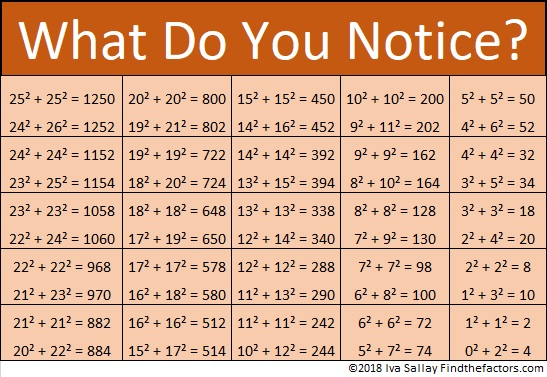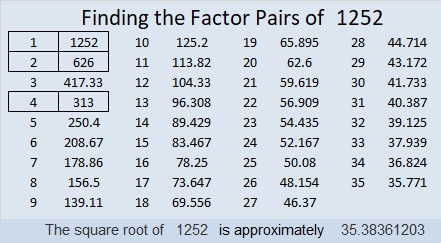# 1252 What Do You Notice?

Remembering that 1250 = 25² + 25² from just a couple of days ago, I was struck when I noticed that 1252 = 24² + 26². I wondered if it was part of a pattern, so I made this chart. What do you think?When I thought about it more, I realized that perhaps it isn’t so remarkable. After all,
(n – 1)² + (n + 1)² = (n² – 2n +1) + (n² + 2n +1) = n² + n² – 2n + 2n + 1 + 1  = 2n² + 2
Nevertheless, I still like the pattern.

Here are some more facts about the number 1252:

• 1252 is a composite number.
• Prime factorization: 1252 = 2 × 2 × 313, which can be written 1252 = 2² × 313
• The exponents in the prime factorization are 2 and 1. Adding one to each and multiplying we get (2 + 1)(1 + 1) = 3 × 2  = 6. Therefore 1252 has exactly 6 factors.
• Factors of 1252: 1, 2, 4, 313, 626, 1252
• Factor pairs: 1252 = 1 × 1252, 2 × 626, or 4 × 313
• Taking the factor pair with the largest square number factor, we get √1252 = (√4)(√313) = 2√313 ≈ 35.38361Because 1252 = 26² + 24², it is the hypotenuse of a Pythagorean triple:
100-1248-1252 calculated from 26² – 24², 2(26)(24), 26² + 24²

100-1248-1252 is also 4 times (25-312-313)